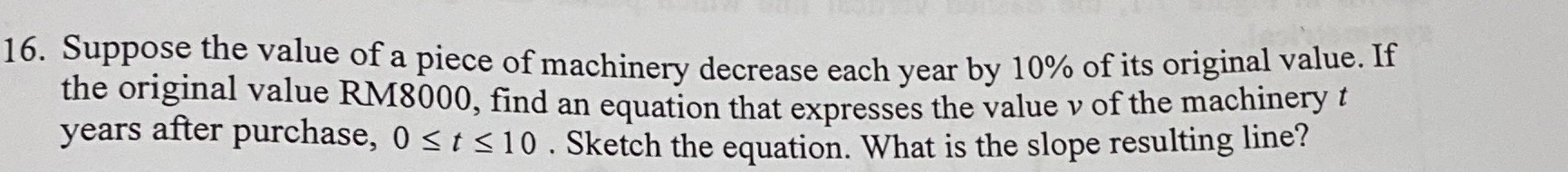### ¿Todavía tienes preguntas de matemáticas?

Pregunte a nuestros tutores expertos
Algebra
Pregunta16. Suppose the value of a piece of machinery decrease each year by $$10 \%$$ of its original value. If the original value RM8000, find an equation that expresses the value $$v$$ of the machinery $$t$$ years after purchase, $$0 \leq t \leq 10$$ . Sketch the equation. What is the slope resulting line?

y=8000$$\times$$0.9$$^{t}$$